# Integration By Parts

### Breaking up a function for easier integration

In differential calculus, you learned how to take the derivative of a product of two functions using the “product rule.” Sometimes, though, you have a function which you can write as a product of two simpler functions, but instead of the derivative, you want to find the integral of this function.

While it's not as simple, or as universal, as the product rule, there is a method to find such integrals. The method is called “integration by parts.”

### The Method

The fundamental equation to use in integration by parts is as follows: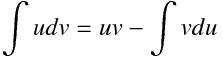What does this mean? Well, it means that if we have two functions u(x), v(x), and we can compute one of the integrals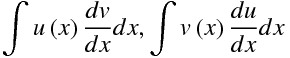then we can also compute the other one. So if we have a function f(x) which we can write in the form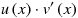, we can often use integration by parts to integrate it. Let's see how it works with an example:

### Example 1

Compute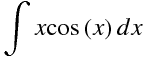### Solution

To use integration by parts, we want to make this integral the integral on the right-hand side of the fundamental equation; in other words, we want to pick some u(x) and v(x) so that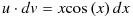.

In fact, if we choose u, we know what dv must be in order to satisfy the equation above; and knowing dv tells us what v(x) is, except for any constant. So we may as well simply choose u(x). One obvious choice, then, is: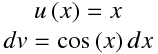Then we have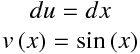So, plugging our inputs into the fundamental equation, we get: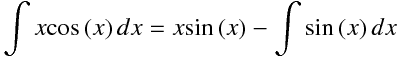But we know how to compute the integral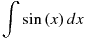. It's just -cos(x). So our final answer is: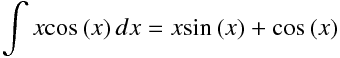### Example 2 (Non-Example)

Integration by parts is as much of an art as it is a science. To use it, you have to figure out the best way to split up an integrand into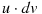. With the wrong choices, the method will often go nowhere.

Let's try to do the above problem again, but this time choose u(x)=cos(x). Then we can quickly find that: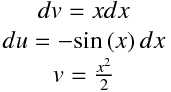If we plug these values into the fundamental equation, we get: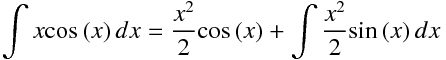But, since we can't evaluate the integral on the right-hand side, we can't simplify this any further!

### The Art Behind The Science

So how do we pick the right u(x) and dv? Well, first of all, you want to be able to integrate whatever you get for dv, since otherwise, you won't be able to compute v(x).

Remember, the goal is to compute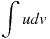by computing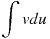, so we often want du to be “simpler” than u. But the best way to learn how to use integration by parts is: Practice!